Fourier Transforming the Damped and Forced Harmonic Oscillator

Here is the second-order ODE for a damped harmonic oscillator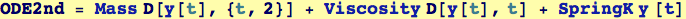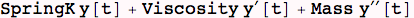Let's  Fourier Transform the left-hand  side of a second-order ODE: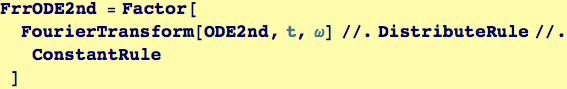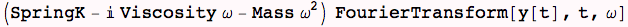And now Fourier Transform the right-hand side for a prototype "forced" oscillator with an arbitary frequency ω0. (i.e., K y''  + η y' + m y = cos(ω0 t)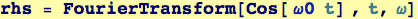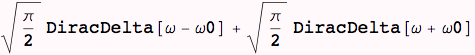Created by Wolfram Mathematica 6.0  (26 November 2007)